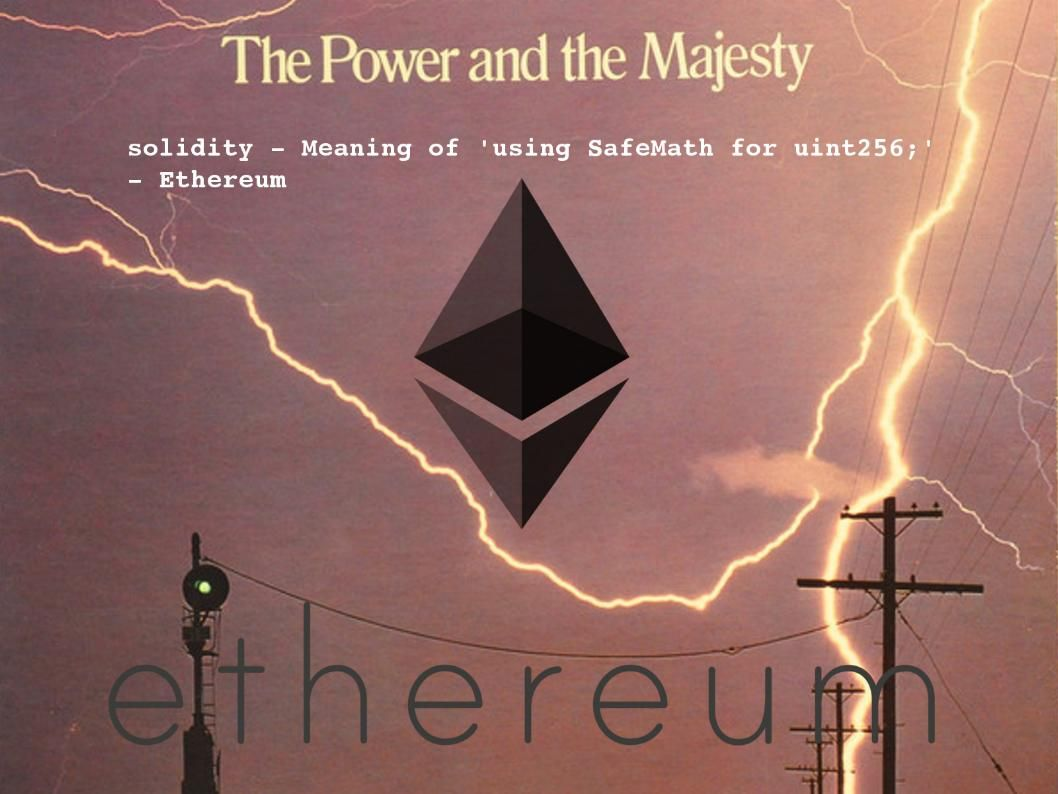# Integer overflow weakness similar kill the Ethereum. But SafeMath to protect from overflows.Integer overflow weakness similar kill the Ethereum – for more details, please see below (url):

Jul 2018 – Integer overflow may killed Ethereum!

But SafeMath to protect from overflows.

c >= a causes integer overflow happen

arithmetic: c=a+b-M (where M = 2**256 is the max unit256 plus one)

If c >=a replace to get a + b -M >=a

Cancelling and recording terms and get b >= M

Result: b is a uint256 and thus b < M

write an algebraic expression in a certain order. We start with the terms that have the largest exponents and work our way down to the constants. Using the commutative property of addition, we can rearrange the terms and put this expression in correct order, like this.

Reference:
Before you evaluate an algebraic expression, you need to simplify it. This will make all your calculations much easier. Here are the basic steps to follow to simplify an algebraic expression:

remove parentheses by multiplying factors
use exponent rules to remove parentheses in terms with exponents
combine like terms by adding coefficients
combine the constants

http://www.math.com/school/subject2/lessons/S2U2L5DP.html

How does the SafeMath library of OpenZeppelin protect your code from integer overflow?

https://ethereum.stackexchange.com/questions/38525/how-does-the-safemath-library-of-openzeppelin-protect-your-code-from-integer-ove?rq=1